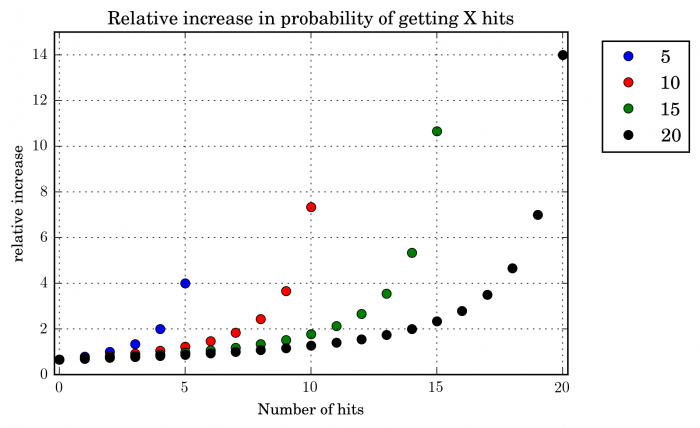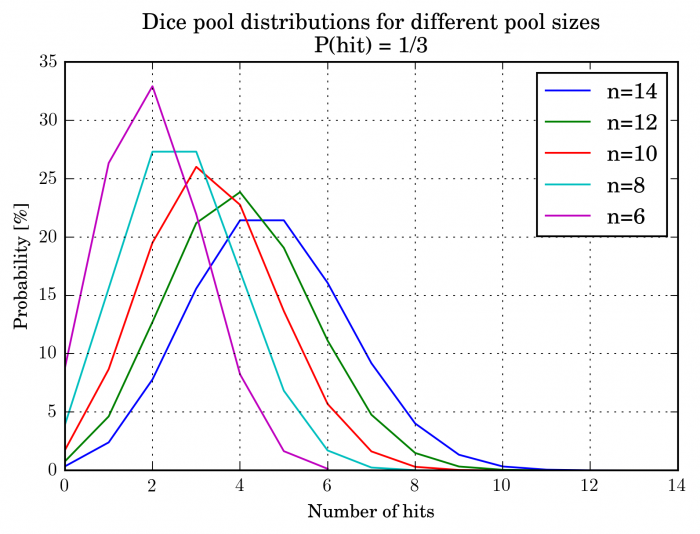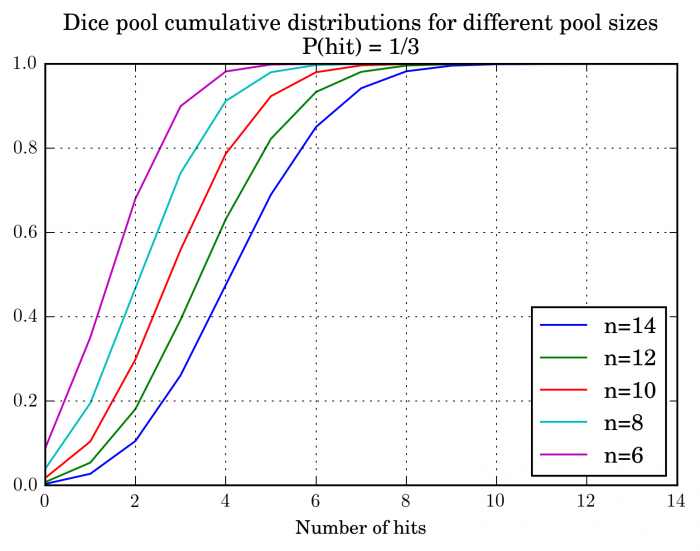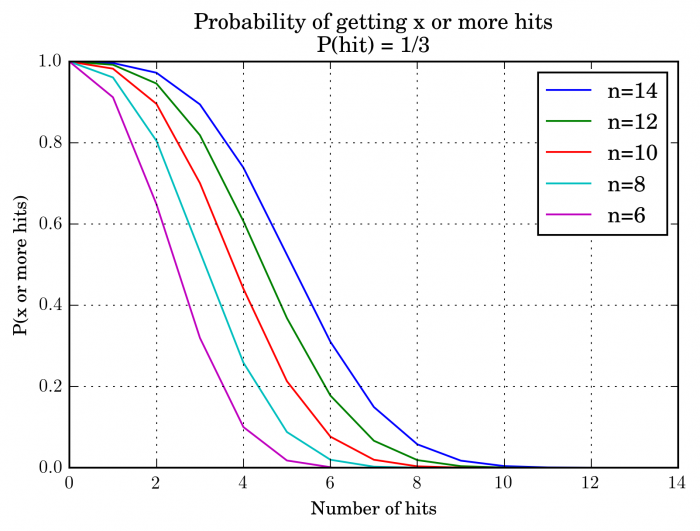Dice math – Part II: Dice pools

In part 1, I covered some properties of a linear dice mechanic using d20 system as an example. Another very common mechanic is the dice pool. The basic idea is that the player rolls multiple similar dice and counts the number of dice that have result equal to or greater than a target number. The number of ‘hits’ is then the result of the roll. Games that use it are numerous: Shadowrun, World of Darkness, Burning wheel, Warhammer (the miniature game!). For example, in Shadowrun, the dice are d6s and the target number is five. So rolling 7 dice and getting numbers 2,3,3,5,6,1,4 would result in two hits. Sometimes there are additional complications, like exploding dice, re-rolls or botches. I’ll cover these on a separate post. The next part is going to be a little math heavy.

A dice pool follows binomial distribution so we can examine its properties analytically. All of this information is readily available in any introductory level probability text book. Also note that I’m using the term ‘hit’ for the success in the individual test (i.e. die) and ‘success’ for a situation, where the number of hits exceeds a threshold.  Given a dice pool size and success probability for each individual dice is p, then the probability of getting k hits is  $$f(k,n,p) = \frac{n!}{k!(n-k)!} p^k (1 - p)^{n-k}$$

Cumulative distribution function, i.e. probability of getting result k or lower, is $$F(k,n,p) = \sum_{i=0}^{k} \frac{n!}{k!(n-k)!} p^k (1 - p)^{n-k}.$$

Mean number of hits is simply $E(hits) = n\cdot p$. So for example, in Shadowrun, if you have a pool of 13 dice, and P(hit) = 1/3, you would expect $13\cdot \frac{1}{3} \approx 4.33$ hits. Another property of the binomial distribution is its variance: $Var(hits) = n\cdot p \cdot (1 - p).$. Note that it stays constant when normalized to pool size.

Then consider, how much the probability of getting k hits increases if you add one die to the pool. Since we are dealing with integers, we can simply consider the following $$\frac{f(k,n+1,p)}{f(k,n,p)},$$

which a little algebra becomes $$\frac{n + 1}{n - k + 1} (1 - p).$$

which gives you the ratio of getting k hits with dice pools n+1 and n. Let’s plot it to get a better idea of how it looks.The idea of the graph is that, for example, given an initial dice pool of, 5 if you get an extra die to the pool, you are about 4 time more likely to score 5 hits than before. Note however, that the initial probability is also very small. The same information could be also gathered from comparing distributions of consecutive dice pools.

Below are two figures illustrating the properties of the distribution: The probability distribution and the cumulative distribution. Note that is the size of the pool.However, these aren’t that interesting from the point of view of game design or player perspective. A more useful form of the second chart inverts it, i.e. while the cumulative distribution gives the fraction of the outcomes that gives k or fewer hits, a more useful property is the probability of getting k or more hits, which is P(k or more hits)=$1 - \sum_{i=0}^{k - 1} \frac{n!}{k!(n-k)!} p^k (1 - p)^{n-k}.$ This gives the following figure:Of course it’s not very easy to do the math in your head while at the table and running the numbers on a computer might be consider a bit of faux pas. Let’s try to formulate some easy to remember rules of thumb. The mean and the median of binomial distribution usually coincide (there are complications, but they are not important for this application), so half of the times you will get more successes than the mean and half the time you will be getting less. This doesn’t get you very far, so consider then the following. The standard deviation is the square root of variance, so for binomial is $\sigma = \sqrt{n\cdot p (1 - p)}$. For example, for Shadowrun, we have $p(1-p) = 2/9 \approx 0.22$ so the standard deviation of your dicepool is $\sigma = 0.47\sqrt{n}$. For example, dice pool of 4 has standard deviation of 0.94 and dice pool of 9 has standard deviation of 1.41. This is a number that you can have a reasonable idea very quickly. Firstly, it is very unlikely (1-2%) to get results three standard deviations above your mean. This basically means that the reasonable number of hits that you should expect is less than $mean + 3\cdot \sigma$. For example, your dice pool is 15. The  mean is 5 plus three time the standard deviation is about 10.4. This means that it is extremely unlikely to get results above this.

I can give the following (very rough) guidelines

• $\sigma \approx 0.47\cdot\sqrt{n}$
• Consider the number $mean + 3\cdot \sigma$ your practical upper limit
• About ~15% of the time you will get  $mean + \sigma$ or more hits
• About 85% of time you will get  $mean- \sigma$ or more hits.

This makes sense, since for larger binomial distribution is approximated well by the normal distribution. However, in practice maybe you should just print figure 3 and stick it next to your character sheet and eyeball your chances. Of course, if you are a game designer, then you have no excuses for being inaccurate and you should calculate the probabilities accurately.

Fortunately, we can deal with addition of results (such as an extended test or teamwork, where multiple individual tests are made) very simply. The joint distribution of variable Z = X + Y, where X and Y follow binomial distributions Bin(n,p) and Bin(m,p) respectively, Z follows binomial distribution Bin(n+m,p). So just add up the dice pools and work with that number. This ignores any special mechanics.

Finally, here are a few tables tabulating properties for different dice pool sizes. I’m again using Shadowrun as an example, so the probability for each die to score a hit is still 1/3. First let’s tabulate probabilities for each possible outcome. I’ll omit a few extreme, improbable values.

 0 1 2 3 4 5 6 7 8 9 10 11 12 4 0.2 0.4 0.3 0.1 0.01 – – – – – – – – 6 0.09 0.26 0.33 0.22 0.08 0.02 0.0 – – – – – – 8 0.04 0.16 0.27 0.27 0.17 0.07 0.02 0.0 0.0 – – – – 10 0.02 0.09 0.2 0.26 0.23 0.14 0.06 0.02 0.0 0.0 0.0 – – 12 0.01 0.05 0.13 0.21 0.24 0.19 0.11 0.05 0.01 0.0 0.0 0.0 0.0 14 0 0.02 0.08 0.16 0.21 0.21 0.16 0.09 0.04 0.01 0.0 0.0 0.0 16 0 0.01 0.05 0.11 0.17 0.21 0.19 0.14 0.08 0.03 0.01 0.0 0.0 18 0 0.01 0.03 0.07 0.13 0.18 0.2 0.17 0.12 0.06 0.03 0.01 0.0 20 0 0 0.01 0.04 0.09 0.15 0.18 0.18 0.15 0.1 0.05 0.02 0.01 22 0 0 0.01 0.03 0.06 0.11 0.16 0.18 0.17 0.13 0.08 0.05 0.02 24 0 0 0 0.02 0.04 0.08 0.12 0.16 0.17 0.15 0.11 0.07 0.04 26 0 0 0 0.01 0.02 0.05 0.09 0.14 0.16 0.16 0.14 0.1 0.06 28 0 0 0 0 0.02 0.04 0.07 0.11 0.14 0.16 0.15 0.12 0.09 30 0 0 0 0 0.01 0.02 0.05 0.08 0.12 0.15 0.15 0.14 0.11

Then a second table will give you the probability to get X or more hits on a given dice pool.

 0 1 2 3 4 5 6 7 8 9 10 11 12 13 14 4 1 0.8 0.41 0.11 0.01 – – – – – – – – – – 6 1 0.91 0.65 0.32 0.1 0.02 0.0 – – – – – – – – 8 1 0.96 0.8 0.53 0.26 0.09 0.02 0.0 0.0 – – – – – – 10 1 0.98 0.9 0.7 0.44 0.21 0.08 0.02 0.0 0.0 0.0 – – – – 12 1 0.99 0.95 0.82 0.61 0.37 0.18 0.07 0.02 0.0 0.0 0.0 0.0 – – 14 1 1 0.97 0.89 0.74 0.52 0.31 0.15 0.06 0.02 0.0 0.0 0.0 0.0 0.0 16 1 1 0.99 0.94 0.83 0.66 0.45 0.26 0.13 0.05 0.02 0.0 0.0 0.0 0.0 18 1 1 0.99 0.97 0.9 0.77 0.59 0.39 0.22 0.11 0.04 0.01 0.0 0.0 0.0 20 1 1 1 0.98 0.94 0.85 0.7 0.52 0.34 0.19 0.09 0.04 0.01 0.0 0.0

Finally, I’ll tabulate some examples for an opposed test giving probabilities for individual outcomes. The first column gives you the dice pools involved in the form player1-player2. I’ll again omit some extreme values.

 -5 -4 -3 -2 -1 0 1 2 3 4 5 5-5 0 0.007 0.037 0.112 0.212 0.263 0.214 0.112 0.036 0.007 0.001 5-10 0.042 0.095 0.165 0.211 0.208 0.145 0.076 0.03 0.007 0.001 0 10-10 0.012 0.032 0.07 0.123 0.167 0.187 0.168 0.121 0.07 0.03 0.012 10-15 0.062 0.103 0.14 0.164 0.166 0.133 0.092 0.053 0.024 0.009 0.002

Let’s try to summarize some of the properties of the system

• Intuitively, the system is easy to understand and the mean results is quickly calculated. However, it is more difficult than in linear system to estimate success probabilities for situations deviating from the mean. Eg. in d20, it is very easy to see that if a roll of 16 is required, that happens 25% of time. However, it isn’t intuitively clear, how often 6 successes or more is rolled with a dice pool of 13 dice.
• Mathematically very neat. Many situations can be solved analytically which could in theory make the system easier to make robust.
• While in theory additions to dice pool are hard to calculate, each additional die gives p additional successes (where is the probability to get a hit on a single die)
• In principle, anyone can beat anyone in opposed tests. However, in practice extreme results are highly unlikely. For example, getting 10 successes on a 10 die dice pool in Shadowrun happens only 0.0017 % of time so about once in 50000 tests.

I will deal with a few special mechanics – such as critical failure, consecutive dice pools and exploding dice – in a separate post. I’ll also consider the situation of changing the target number. I’ll link it here when it is available.

Warning: Error while sending QUERY packet. PID=231561 in /home/trivialh/public_html/wp-includes/wp-db.php on line 2056